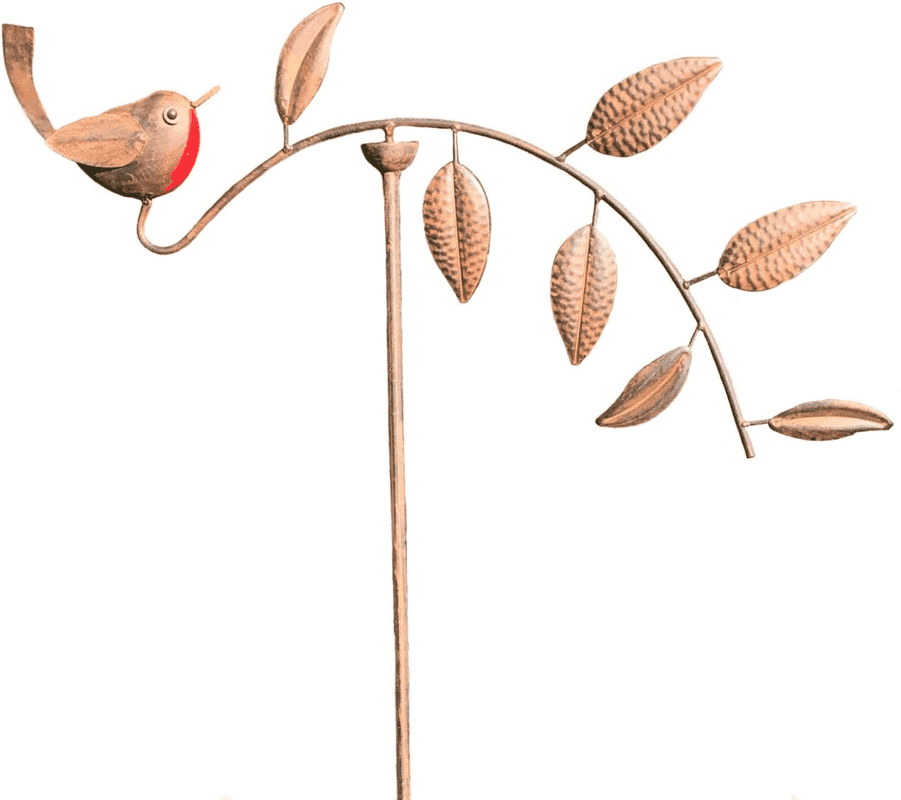# Torque of an electric motor

Gold Member
Hi guys,
In my head, torque has also been associated with a bare SCALAR physical quantity given by the formula ##torque=rFsin\theta## which caused a rigid body to spin around its axis.
Now, studying magnetism, I find out that torque is a VECTOR, which magnitude is indeed given by ##rFsin\theta## and with the direction of the axis and pointing upwards/downwards depending on if the body is spinning clockwise/counterclockwise.
Now, my question is: if torque lets a body spin, how could it be a vector coincident with the axis of rotation? Which physical meaning has its vectorial nature?

Delta2
Homework Helper
Gold Member
Mathematically the torque ##\vec{T}## is the cross product of the force ##\vec{F}##and the position vector ##\vec{r}## of the force. In vector notation it is ##\vec{T}=\vec{r}\times\vec{F}##. You can check more about the cross product on these pages
https://en.wikipedia.org/wiki/Cross_product
https://en.wikipedia.org/wiki/Right-hand_rule
I know if you thinking in 2 dimensions you probably think like "all I want to know is if the torque is clockwise or counterclockwise", because in 2D the axis of rotation is taken for granted and is usually perpendicular to the plane of the page, however when we try to work in 3 dimensions, it is important why the torque give us the axis of rotation under which this torque will rotate the body if it was the total torque. That's it's physical meaning, it gives us the axis of rotation.

Last edited:
••Lnewqban and greg_rack
Gold Member
Mathematically the torque ##\vec{T}## is the cross product of the force ##\vec{F}##and the position vector ##\vec{r}## of the force. In vector notation it is ##\vec{T}=\vec{r}\times\vec{F}##. You can check more about the cross product on these pages
https://en.wikipedia.org/wiki/Cross_product
https://en.wikipedia.org/wiki/Right-hand_rule
I know if you thinking in 2 dimensions you probably think like "all I want to know is if the torque is clockwise or counterclockwise", because in 2D the axis of rotation is taken for granted and is usually perpendicular to the plane of the page, however when we try to work in 3 dimensions, it is important why the torque give us the axis of rotation under which this torque will rotate the body if it was the total torque. That's it's physical meaning, it gives us the axis of rotation.
Thanks, got it!

A.T.
Now, my question is: if torque lets a body spin, how could it be a vector coincident with the axis of rotation? Which physical meaning has its vectorial nature?

••greg_rack and etotheipi
etotheipi

This is nice! When I was a bit smaller, something that confused me was that you come across these sorts of ornaments in garden centres and whatnotthat are in stable equilibrium. Without that small vertical piece, tilting the thing on the top by an arbitrary angle would contract the perpendicular distances of the centres of mass of either side to the axis by the same fraction, and the ornament would remain in its new equilibrium position (i.e. neutral equilibrium). But the vertical piece destroys that symmetry, as demonstrated in your video, and ensures that there is actually a restoring torque!

•Lnewqban and greg_rack